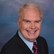# Math Solutions: Pump Performance And Efficiency CalculationsBy Daniel Theobald, "Wastewater Dan"

Water Online’s “Math Solutions,” presented by wastewater consultant and trainer Dan Theobald (“Wastewater Dan”), instructs operators on pump performance and efficiency calculations.

Pump performance and efficiency calculations, whether for certification exams or for operating your plant, may require various head pressure calculations, different horsepower calculations, or certain efficiency calculations.

Depending on your required math solution pertaining to pumps, calculations and unit of expression require explicit formulas.

Some common pump performance and efficiency terms are defined, and their calculations are accessible below:

Definitions and Math Solutions for various pump performance efficiency calculations

Suction Lift Head Pressure-Ft.: The distance from where the liquid is taken to the centerline of the pump

Discharge Head Pressure-Ft.: The distance in feet that the liquid leaves a pump

Total Dynamic Head (TDH) Pressure-Ft: The total equivalent height in feet that a fluid is to be pumped, taking into account friction losses in the pipe

Water Horsepower: A ratio of water horsepower out divided by the mechanical horsepower into the pump

Calculate-Water Horsepower:

Brake Horsepower: The horsepower available at the output shaft of a motor

Calculate-Brake Horsepower:

Wire-to-Water Horsepower: Wire-to-water (electrical or motor) horsepower is calculated by dividing brake horsepower by motor efficiency

Calculate-Wire-to-Water Horsepower:

Wire-to-Water Efficiency Percentage (%): The combined efficiency of a motor and pump system, calculated by multiplying the motor efficiency by the pump efficiency

Calculate-Wire-to-Water Efficiency Percentage (%):

Complete playlist of Wastewater Dan’s Math Solutions

This is the Pump Performance And Efficiency Calculations presentation in my series of “Math Solutions.” If you have specific wastewater math queries, please submit a question.